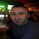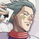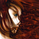# UsdJpy - trading with pivot points and pivot trend lines

FX:USDJPY   U.S. Dollar / Japanese Yen
731 views
731
Yen is sitting on the daily trend line below the daily pivot - a break below the daily trend should bring a challenge to the break out of the cup and handle play which is also demand. A break above the daily pivot will bring price back to R3. The chart contains the source code for auto pivot trend lines so they do not have to be drawn by hand. If you want the indicator make the chart yours and you should be able to load the indicator into your own base.
Tradunity.comMany thanks to all of you for the contributions :)
Replyfxarchi
on the chart there is a star symblol click under it the one with the 3 circles
Replystudy("Kate fib Pivots", overlay = true,precision=1)
TimeFrame = input(title="Timeframe", type=resolution, defval="1640")
Trendline =input(true)

High = security(tickerid,TimeFrame, high)
Low = security(tickerid,TimeFrame, low)
Close = security(tickerid,TimeFrame, close)

PP = ( High + Low + Close ) / 3
R3 = PP + 1.000 * (High - Low)
R2 = PP + 0.618 * (High -Low)
R1 = PP + 0.382 * (High -Low)
S1 = PP - 0.382 * (High -Low)
S2 = PP - 0.618 * (High - Low)
S3 = PP - 1.000 * (High - Low)

trend1=PP != PP ? PP : na
trend2=PP != PP ? PP : na
plot(Trendline?trend1:na,color=black, linewidth = 2)
plot(Trendline?trend2:na,color=black, linewidth = 2)

plot(PP, color=red, title="PP", style = circles, linewidth = 2)
plot(R1, color=blue, title="R1", style = circles, linewidth = 3)
plot(R2, color=purple, title="R2", style = circles, linewidth = 1)
plot(R3, color=fuchsia, title="R3", style = circles, linewidth = 1)
plot(S1, color=blue, title="S1", style = circles, linewidth = 3)
plot(S2, color=purple, title="S2", style = circles, linewidth = 1)
plot(S3, color=fuchsia, title="S3", style = circles, linewidth = 1)
Replykate25
trend1=PP != PP ? PP : na
trend2=PP != PP ? PP : na

//the are to read the previous pivot values and compare
Replyglaz
i see, there is a problem when pasting scipts at the chat parts of the code get cut off, best way is to "make it mine" as Kate said.
ReplyGlaz wrote the indicator - if you use the indicator pay more attention to the trend line drawn end to end of the pivot point - thank you so much Glaz for creating the script - the glory is all yours and the community has another indicator
Reply